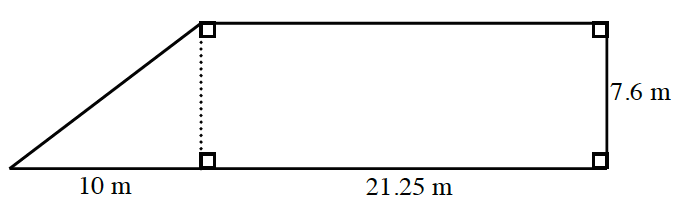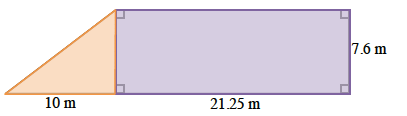### Home > CC2MN > Chapter 9 > Lesson 9.1.2 > Problem9-23

9-23.

Find the area of the shape at right. Show your steps.Start by finding the areas of each separate shape.

$\text{Area of Triangle: } \frac{1}{2}\text{ (base)(height)}$
$\text{Area of Rectangle}:(\text{length})(\text{width})$After you find the area of each separate shape, add the two areas together to find the total area of the shape.

Total Area: $199.5 \text{ m}^2$
Make sure you know how to get this answer.